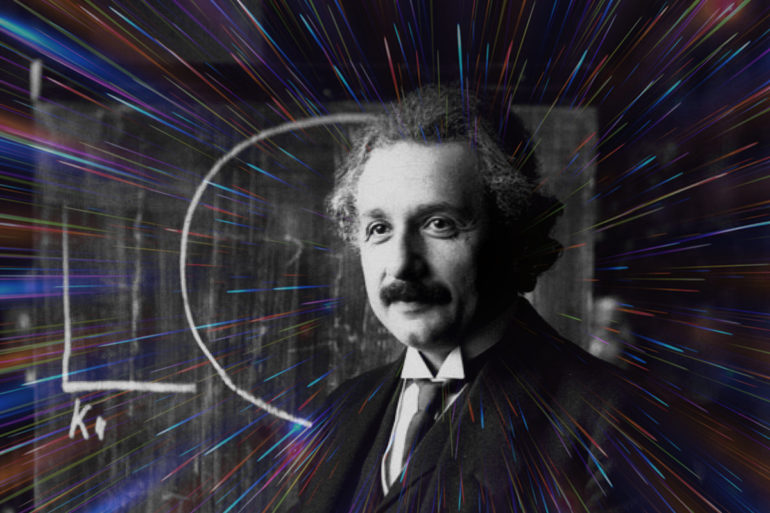## What does Einstein’s E=mc2 mean?

What does Einstein’s famous equation E= mc2 actually mean? What equals what what? Early in the nineteenth century, Albert Einstein, while working as a clerk in a patent office, was inspired to propose a relationship between mass (the m), and energy (the E). His E=mc2 equation just states, in basic mathematical terms, that there is…